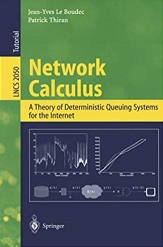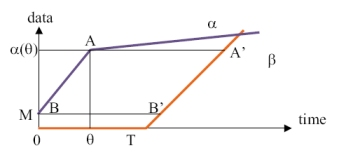# The Network Calculus BookThe Network Calculus book by Jean-Yves Le Boudec and Patrick Thiran is available for free download: pdf.

The book is also available as Springer-Verlag Lecture Notes on Computer Science number 2050.

The source files are available here.

### What is Network Calculus ?

Network Calculus is a collection of results based primarily on min-plus algebra, which applies to deterministic queuing systems found in communication networks. It can be used to understand:• what is a T-SPEC or arrival curve constraint;
• how to compute delay and backlog bounds in deterministic or time sensitive networks;
• why re-shaping delays can be ignored in shapers or spacer-controllers;
• a common model for schedulers;
• and much more.

### Tutorials

• "A Short Introduction to Network Calculus", pdf, IMAG, Grenoble, Nov 12, 2019,
• "A Long Introduction to Network Calculus", pdf, video, Dagstuhl Seminar on Analysis, Design, and Control of Predictable Interconnected Systems, March 4 and EPFL April 5, 2019,
• A Short Course (2 to 3 hours) ppt pdf
• EPFL Doctoral School 2005 click here